« Unfavourable loadings and envelopes of stresses - deflections Example 2 » Example 1 (project <Β_422-1>)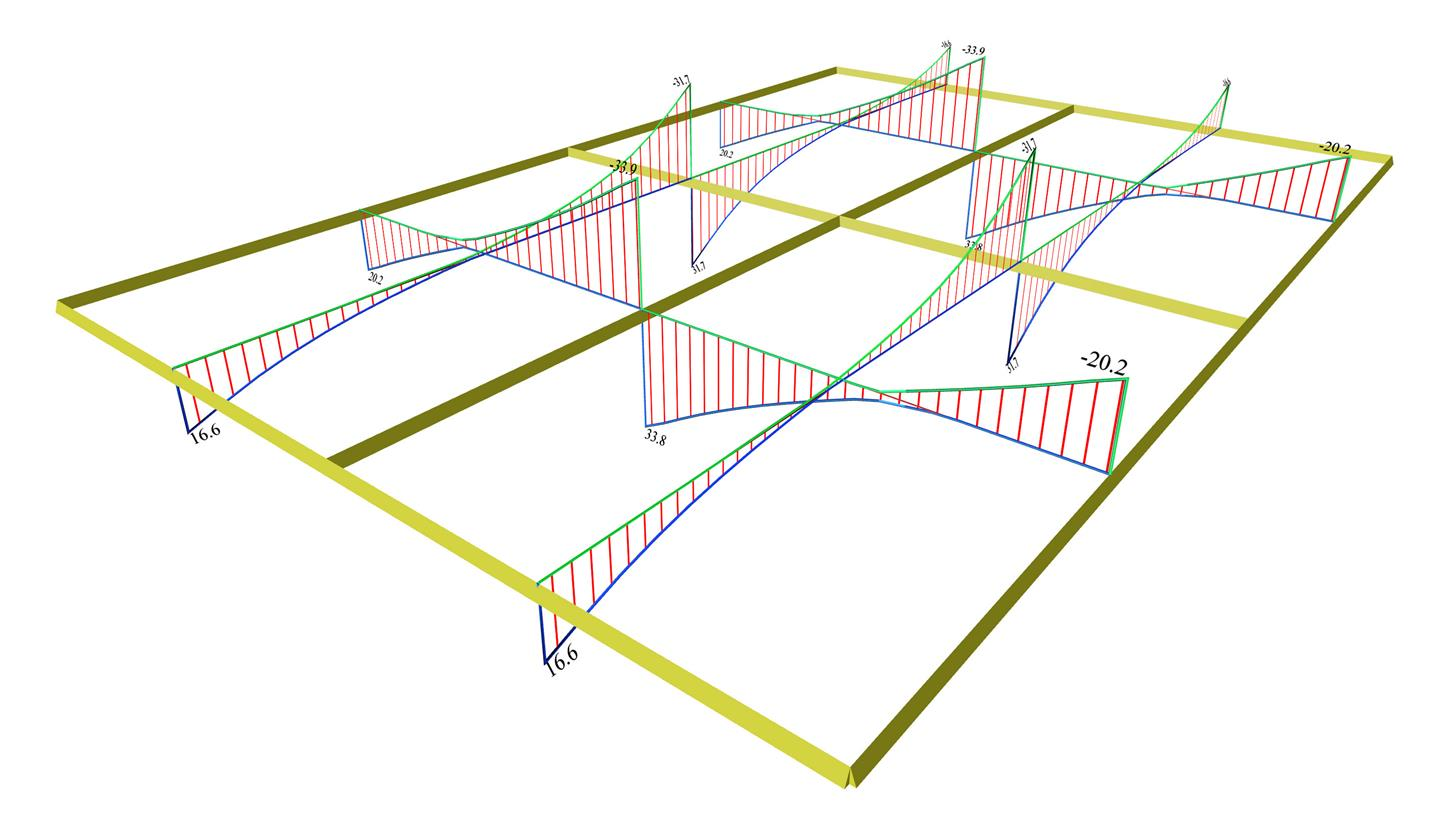Figure 4.2.3-3: Shear forces envelopes Figure 4.2.3-3: Shear forces envelopes The most unfavourable shear forces are equal to: x direction: Vxr= 20.2 (18.8 [*]NoteThe respective results corresponding to global loading are shown in brackets. , Vx,erm=-33.8 (-33.3) [kN] y direction: Vyr=16.6 (15.1), Vy,erm=-31.7 (-29.0) [kN]Figure 4.2.3-4: Front view of 3D shear force diagrams, corresponding to the envelope of [Vx] Figure 4.2.3-4: Front view of 3D shear force diagrams, corresponding to the envelope of [Vx]Figure 4.2.3-5: Side view of 3D shear force diagrams, corresponding to the envelope of [Vy] Figure 4.2.3-5: Side view of 3D shear force diagrams, corresponding to the envelope of [Vy] Note that although the live load has a relatively high value, the differences between the shear forces do not exceed 8%.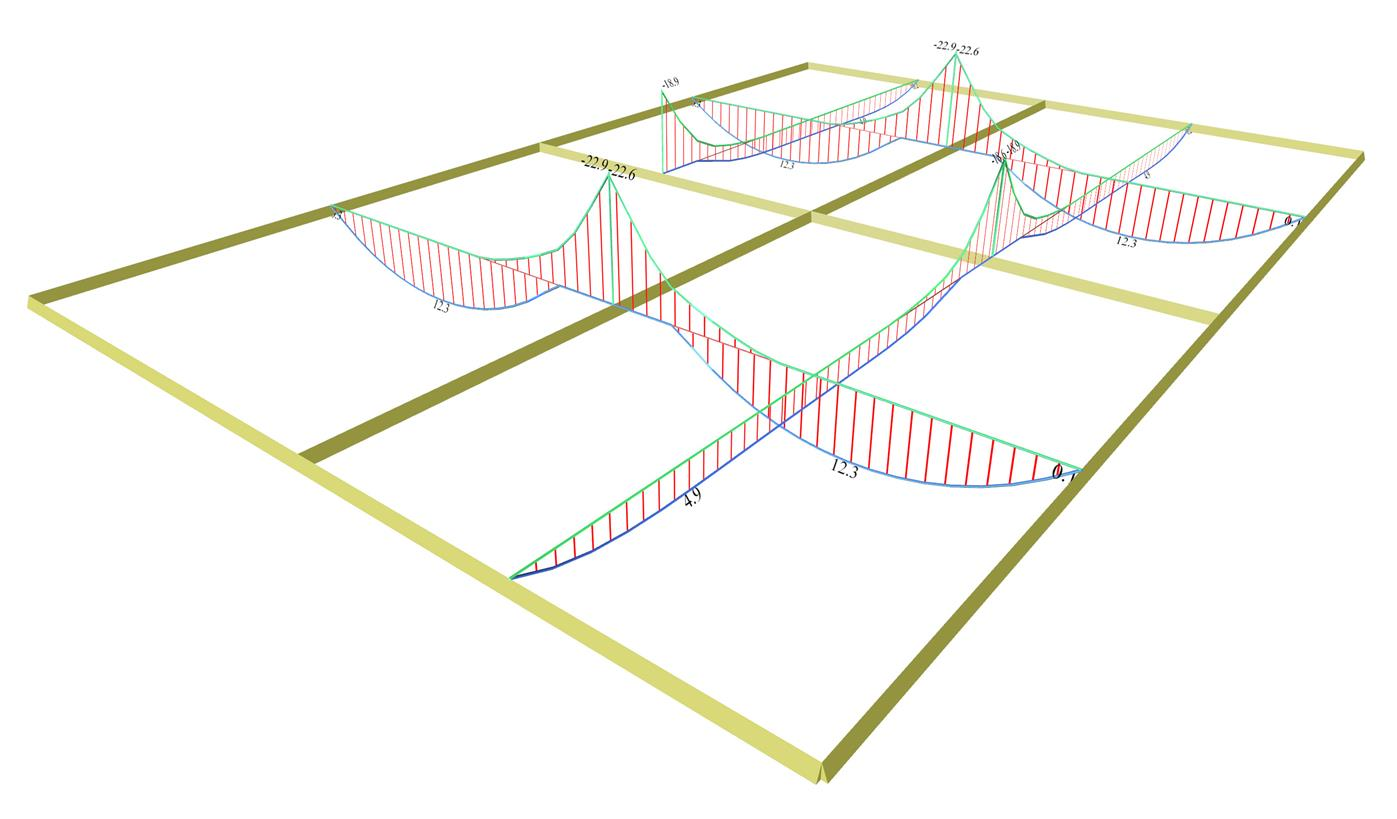Figure 4.2.3-6: Bending moment envelopes Figure 4.2.3-6: Bending moment envelopes The most unfavourable bending moments are equal to: x direction: Mx=12.3 (10.8) [*]NoteThe respective results corresponding to global loading are shown in brackets. , Mx,erm=-22.8 (-22.0) [kNm] y direction: My=4.9 (4.1), My,erm=-18.8 (-16.3) [kNm]Figure 4.2.3-7: Front view of 3D bending moment diagrams, corresponding to the envelope of [Mx] Figure 4.2.3-7: Front view of 3D bending moment diagrams, corresponding to the envelope of [Mx]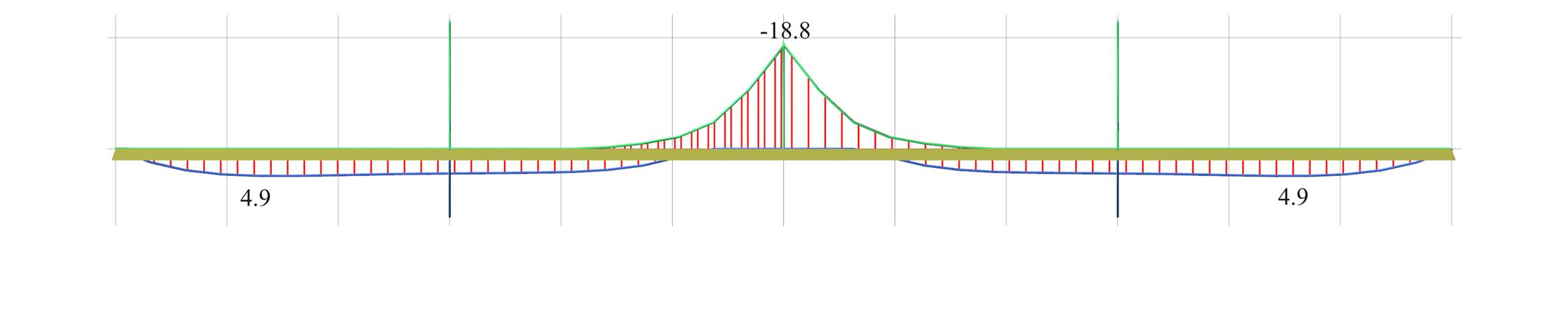Figure 4.2.3-8: Side view of 3D bending moment diagrams, corresponding to the envelope of [My] Figure 4.2.3-8: Side view of 3D bending moment diagrams, corresponding to the envelope of [My] Note that although the live load has a relatively high value, the differences between the bending moments are small and less than15%.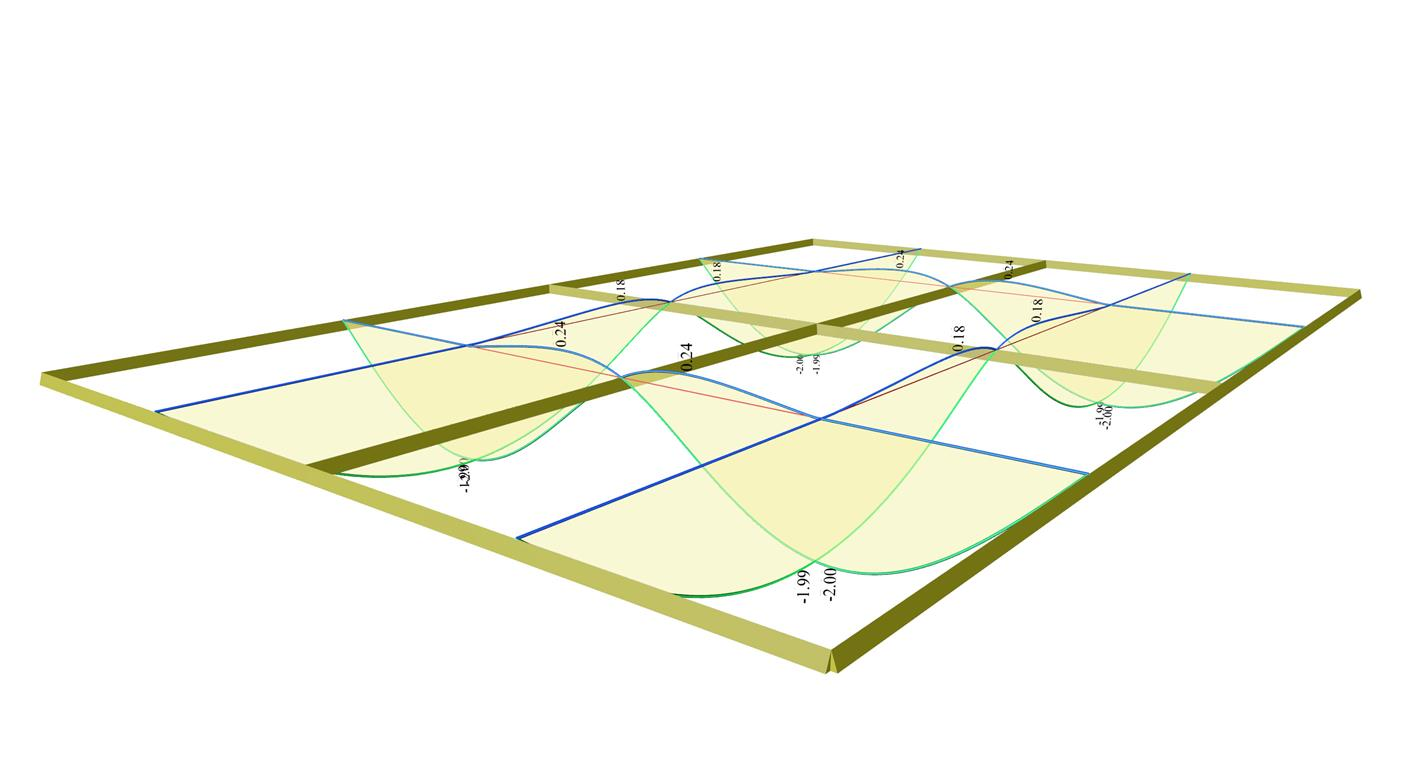Figure 4.2.3-9: Deflection envelopes Figure 4.2.3-9: Deflection envelopes The largest slab deflection is equal to y=-2.00 (-1.53) [*]NoteThe respective results corresponding to global loading are shown in brackets. mm and y=+0.24 mm. It is concluded that the slab is lifted, which is in contrast with the responseof the global loading case, due to symmetry of course.Figure 4.2.3-10: Front view of 3D deflection diagrams Figure 4.2.3-10: Front view of 3D deflection diagrams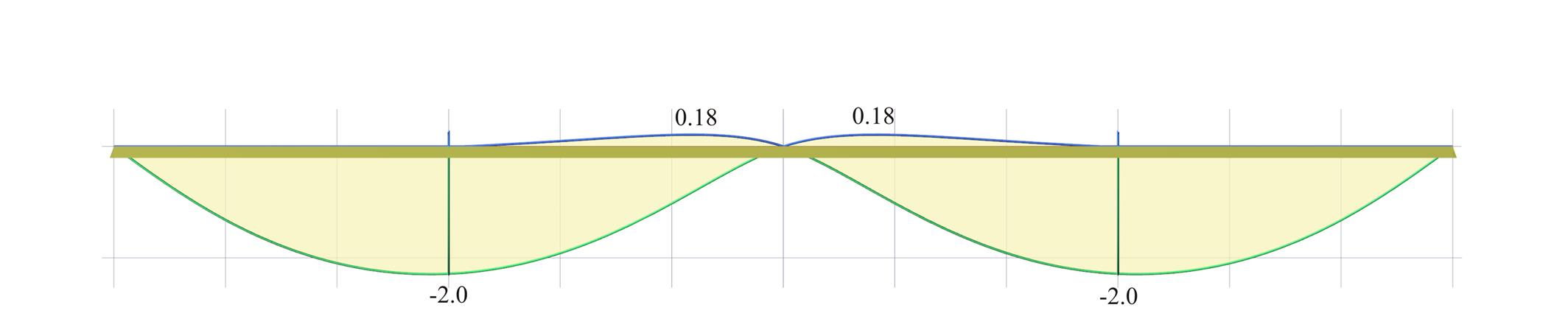Figure 4.2.3-11: Side view of 3D deflection diagrams Figure 4.2.3-11: Side view of 3D deflection diagrams Notice that the deflections are as high as 30% while opposite sign deformations also arise (blue lines). « Unfavourable loadings and envelopes of stresses - deflections Example 2 »